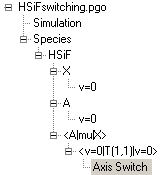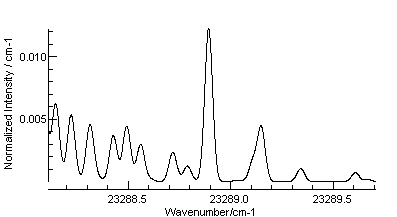# Axis Switching

To simulate axis switching transitions, add an "Axis Switching" object under a transition moment object; currently it is implemented for Cartesian or Spherical transition moments for asymmetric tops. The rotation between the axes in the two states is specified by the three Euler angles:

## Parameters

 Chi Axis switching angle χT (degrees) Theta Axis switching angle θT (degrees) Phi Axis switching angle φT (degrees)

The basic theory was originally described in Hougen and Watson (1964). The implementation here is more general, using an exact evaluations of the Wigner D matrix and not assuming that K is a good quantum number, rather than the approximations used by Hougen and Watson. It is also implemented for multiphoton transitions. More general results can be found in Dixon and Wright (1985) (for rotation about a single axis, θ) and Huet at al (1990) (including all thee axes). The formula used relies on the fact that the wavefunctions have the form of Wigner D matrices (Brown and Howard, 1976):
|NMK> = [(2J+1)/(8π2)]½DMK N(ω)*
and rotating the the Wigner D matrices though the axis switching angles (χT, θT, φT) produces a sum over Wigner D matrices with the same N and M:
DMK N(ω') = ΣK' DK'K NT, θT, φT) DMK' N(ω)
Now consider an arbitrary transition dipole moment, <N'M'K'; ω'| T | NMK; ω>, where the body fixed axes in the bra and ket are different and ω' represents the angle between the space and body fixed angles used for the bra and ω for the ket. Using the equation above we can transform the bra the bra to use the axis system of the ket:
<N'M'K'; ω'| = [(2J+1)/(8π2)]½DM'K' N'(ω') = [(2J+1)/(8π2)]½ ΣK" DK"K' N'T, θT, φT) DM'K" N'(ω) = ΣK" DK"K' N'T, θT, φT) <N'M'K"; ω|
The transition moment can thus be expressed in terms of rotational matrix elements all in the same axis system:
<N'M'K'; ω'| T |NMK; ω> = ΣK" DK"K' N'T, θT, φT)<N'M'K"; ω| T |NMK; ω>
and the transition dipole matrix elements <N'M'K"; ω| T | NMK; ω> are now the standard ones used by PGOPHER.

## Example - HSiF

The datafile HSiFswitching.pgo produces the simulation shown below, which reproduces the right hand 2/3 of figure 3c of Dixon and Wright (1985). Note the axis switching angle, θT, set to 2.3 in the "Axis Switch" object under the T(1,1) Spherical transition moment. To reproduce figure 3b, with no axis switching, set the angle to 0. Note the choice of axes is important - θT is the rotation about an axis perpendicular to the axis defining K.## References

• "An approach to the anomalous commutation relations of rotational angular momenta in molecules", J M Brown and B J Howard, Mol Phys 31 1517 (1976).
• "The rotational analysis of a Doppler-limited A1A" - X1A' fluorescence excitation spectrum of HSiF at 430 nm", R N Dixon and N G Wright, Chem Phys Lett, 117 280 (1985).
• "The A electronic state of acetylene: geometry and axis-switching effects.", T R Huet, M Godefroid and M Herman, J Mol Spec, 144 32 (1990).
• "Anomalous rotational line intensities in electronic transitions of polyatomic molecules: Axis-switching", J T Hougen and J K G Watson, Can J Phys, 43 298 (1965).